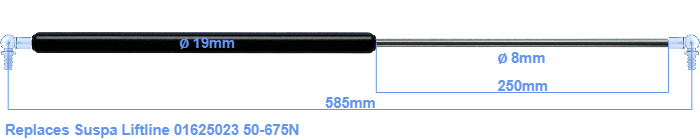# Replacement for Suspa Liftline 01625023 50-675N

\$42.45

Out Of Stock until approx. June 29, 2021

Replacement gas spring for the Suspa Liftline 01625023 50-675 Newton. This fits a (possibly already present) ball with a diameter of 10mm. A corresponding ball is included. Brand: HAHN, a Stabilus company.
 Force Choose an option50 Newton60 Newton80 Newton100 Newton120 Newton140 Newton150 Newton160 Newton180 Newton200 Newton220 Newton240 Newton250 Newton260 Newton280 Newton300 Newton320 Newton340 Newton350 Newton360 Newton380 Newton400 Newton420 Newton440 Newton450 Newton460 Newton480 Newton500 Newton520 Newton540 Newton550 Newton560 Newton580 Newton600 Newton620 Newton640 Newton650 Newton660 Newton675 NewtonClear
This gas spring is also known as 16-2 016 25023, 16-2-294-253,5-A246-B246.This gas spring has a cylinder diameter of 19 mm. The diameter of the rod totals 8 mm. The stroke (the retracting part) has a length of 250 mm. The total length of this replacement is 585 millimeter. Note: this is the total length between the rotation points of the two mounting parts. The distance from thread to thread (without mounting parts) is 545 mm. This replacement gas spring (01625023) has a force of 50-675 Newton (N). This gas spring can replace a Suspa Liftline gas spring, but is not an orignal Suspa Liftline one. HAHN Gasfedern, a Stabilus subsidiary, is responsible for the production of this replacement gas spring. The gas spring has the brand HAHN. Nevertheless, the dimensions and force are equal.
Category: